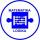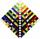# AP - simple

Find the first ten members of the sequence if a11 = 132, d = 3.

Result

a10 =  129
a9 =  126
a8 =  123
a7 =  120
a6 =  117
a5 =  114
a4 =  111
a3 =  108
a2 =  105
a1 =  102

#### Solution:Leave us a comment of example and its solution (i.e. if it is still somewhat unclear...):

Showing 0 comments:Be the first to comment!## Next similar examples:

1. AP - simpleDetermine the first nine elements of sequence if a10 = -1 and d = 4
2. 6 termsFind the first six terms of the sequence. a1 = 7, an = an-1 + 6
3. Nineteenth memberFind the nineteenth member of the arithmetic sequence: a1=33 d=5 find a19
4. AS - sequenceWhat are the first ten members of the sequence if a11=22, d=2.
5. SequenceWrite the first 6 members of these sequence: a1 = 5 a2 = 7 an+2 = an+1 +2 an
6. SequenceWrite the first 7 members of an arithmetic sequence: a1=-3, d=6.
7. Sequence 3Write the first 5 members of an arithmetic sequence: a4=-35, a11=-105.
8. Sequence 2Write the first 5 members of an arithmetic sequence a11=-14, d=-1
9. Sum of membersWhat is the sum of the first two members of the aritmetic progression if d = -4.3 and a3 = 7.5?
10. SeatsSeats in the sport hall are organized so that each subsequent row has five more seats. First has 10 seats. How many seats are: a) in the eighth row b) in the eighteenth row
11. AS sequenceIn an arithmetic sequence is given the difference d = -3 and a71 = 455. a) Determine the value of a62 b) Determine the sum of 71 members.
12. SequenceBetween numbers 1 and 53 insert n members of the arithmetic sequence that its sum is 702.
13. TreesA certain species of tree grows an average of 0.5 cm per week. Write an equation for the sequence that represents the weekly height of this tree in centimeters if the measurements begin when the tree is 200 centimeters tall.
14. SeriesYour task is express the sum of the following arithmetic series for n = 14: S(n) = 11 + 13 + 15 + 17 + ... + 2n+9 + 2n+11
15. Three unknownsSolve the system of linear equations with three unknowns: A + B + C = 14 B - A - C = 4 2A - B + C = 0
16. AverageIf the average(arithmetic mean) of three numbers x,y,z is 50. What is the average of there numbers (3x +10), (3y +10), (3z+10) ?
17. Theorem proveWe want to prove the sentense: If the natural number n is divisible by six, then n is divisible by three. From what assumption we started?